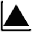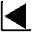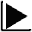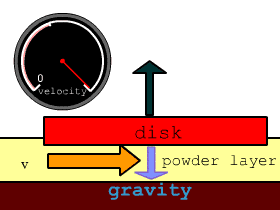Measurement of Friction Coefficient on a Powder Layer and Fluid Dynamic Study## Discussion 1

It was confirmed that friction between solid bodies can be lowered by the presence of a powder layer under certain conditions (Phase B). In this section, we discuss the mechanism for producing  low friction coefficient in phase B.

In the control run where the powder layer was pressed, the speed curve of the disk did not show the typical change observed in  Major results 2 and phase B was not observed. Because fluffy powder fluidizes more easily than pressed powder, this result indicates that the phase B requires high fluidity of the powder.

Since it was observed in phase B that the powder layer remained fluffy after the disk passed over, the disk most likely "floated" over the fluidized powder layer.

 Suppose that this flotation causes a drop in friction. The source of this buoyancy then comes into question because the disk is much heavier than the powder.  The experimental results showed that when the velocity of the disk is low, the disk rapidly decelerate while sweeping out the powder layer (Phase C). This suggests that there is a critical speed at which the disk can float. When the speed is lower than this critical, the disk sinks (Fig. 22). This speed is termed the critical speed of flotation in this paper. In the following section, we will  try to identify the mechanism of flotation and discuss whether it can explain the existence of the critical speed and provide its value.Fig. 22. Schema of  a floating critical speed drawn in a fixed coordinate system located on the disk. The disk is floated by a force provided by some mechanism existing in the powder layer corresponding to the relative velocity. If the speed of the disk become smaller than that critical speed, the disk will sink.
 Measurement of Friction Coefficient on a Powder Layer and Fluid Dynamic Study